Author: Oscar Cronquist Article last updated on July 22, 2022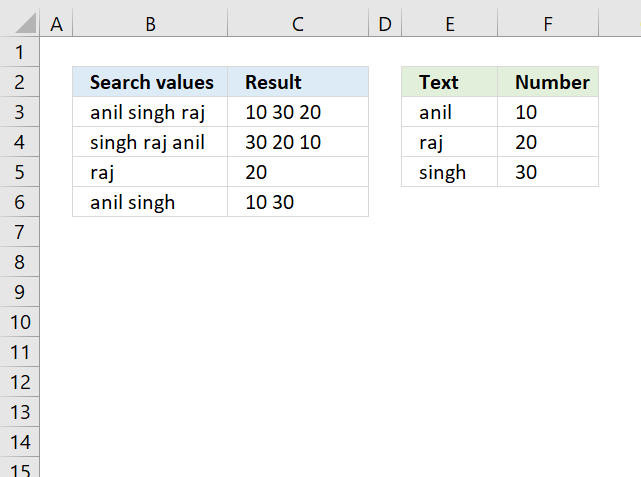This article demonstrates formulas and a UDF that searches for values in a table based on concatenated values and returns the corresponding values also concatenated.

The example image above shows "anil singh raj" in cell B3, the UDF and formulas split the string in substrings and search for each sub-string in cell range E3:E5. If a match is found the corresponding value in cell range F3:F5 is returned.

The result values are concatenated if multiple values are matching values in cell range E3:E5. "anil" is found in cell E3, the corresponding value is 10. "singh" is found in cell E5, the corresponding value is 30. Value "raj" is found in cell E4 and the corresponding value in cell F4 is 20. The value returned in cell C3 is 10 30 20.

## 1. Question

I have
A1(anil singh raj)
It can be anything Like
A1(singh raj anil)
I want return value in
B1 (10 30 20)
Or
B1(30 20 10)
Or
Lookup array is
D1(anil) E1(10)
D2(raj) E2(20)
D3(singh) E3(30)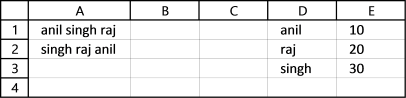I have made a small custom function to split the search string and get the values you are looking for.

## 2. User Defined Function Syntax

SearchValues(str, search_col, return_col)

str - Search string

search_col - lookup column

return_col - values to return

## 3. UDF FormulaFormula in cell C3:

=SearchValues(B3, \$E\$3:\$E\$5, \$F\$3:\$F\$5)

## 4. User Defined Function - VBA

```'Name Function
Function SearchValues(str As Range, search_col As Range, return_col As Range)

'Dimension variables and declare data types
Dim j As Long, i As Long

'Split text in variable str based on a space character
arr = Split(str, " ")

'Save the number of rows in range variable search_col to variable j
j = search_col.Rows.CountLarge

'Iterate through all array values in variable arr
For Each Vl In arr

'Go from 1 to number stored in variable j
For i = 1 To j

'If ... Then statement
'Check if value in range variable search_col is equal to variable Vl
'Concatenate corresponding value in return_col with result variable if true
If search_col.Cells(i, 1) = Vl Then result = result & return_col.Cells(i, 1) & " "
Next i
Next Vl

'Return string stored in result to worksheet
SearchValues = result
End Function
```

## 5. Where to put the VBA code?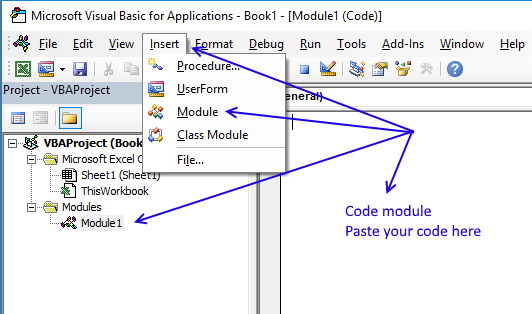You need to copy the code above and paste it to a code module, detailed instructions below.

1. Open vb editor (shortcut keys: Alt+F11)
2. Insert a new module
3. Paste code to code module
4. Exit vb Editor

Note, save your workbook as a macro-enabled workbook *.xlsm to keep the code attached to your workbook.

## 6. Excel 2019 Formula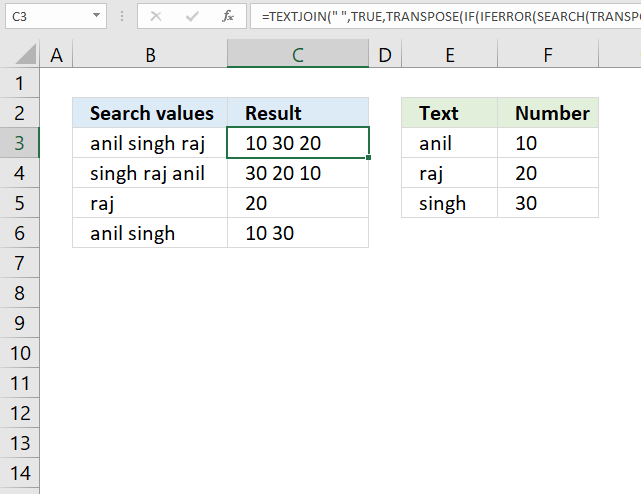Array formula in cell C3:

=TEXTJOIN(" ", TRUE, TRANSPOSE(IF(IFERROR(SEARCH(TRANSPOSE(FILTERXML("<A><B>"& SUBSTITUTE(B3, " ", "</B><B>") & "</B></A>", "//B")), \$E\$3:\$E\$5), 0), \$F\$3:\$F\$5, "")))

### 6.1 Explaining formula in cell C3

#### Step 1 - Insert XML tags

The SUBSTITUTE function replaces a specific text string in a value. We need to replace the delimiting character to xml tags in order to split the strings into an array.

SUBSTITUTE(textold_textnew_text, [instance_num])

"<A><B>"& SUBSTITUTE(B3, " ", "</B><B>") & "</B></A>"

becomes

"<A><B>"&"anil</B><B>singh</B><B>raj"& "</B></A>"

and returns

"<A><B>anil</B><B>singh</B><B>raj</B></A>"

#### Step 2 - Split string into substrings

The FILTERXML function extracts specific values from XML content by using the given xpath. You can use this function to split a string into substrings.

FILTERXML("<A><B>"& SUBSTITUTE(B3, " ", "</B><B>") & "</B></A>", "//B")

becomes

FILTERXML("<A><B>anil</B><B>singh</B><B>raj</B></A>", "//B")

and returns {"anil";"singh";"raj"}.

#### Step 3 - Convert a vertical range to a horizontal range, or vice versa

The TRANSPOSE function allows you to convert a vertical range to a horizontal range, or vice versa.

TRANSPOSE(FILTERXML("<A><B>"& SUBSTITUTE(B3, " ", "</B><B>") & "</B></A>", "//B"))

becomes

TRANSPOSE({"anil"; "singh"; "raj"})

and returns {"anil", "singh", "raj"}.

#### Step 4 - Search for each value in the array in cell range \$E\$3:\$E\$5

The SEARCH function returns a number representing the position of character at which a specific text string is found reading left to right.

SEARCH(find_text,within_text, [start_num])

SEARCH(TRANSPOSE(FILTERXML("<A><B>"& SUBSTITUTE(B3, " ", "</B><B>") & "</B></A>", "//B")), \$E\$3:\$E\$5)

becomes

SEARCH( {"anil", "singh", "raj"}, \$E\$3:\$E\$5)

becomes

SEARCH( {"anil", "singh", "raj"}, {"anil";"raj";"singh"})

and returns {1, #VALUE!, #VALUE!; #VALUE!, #VALUE!, 1; #VALUE!, 1, #VALUE!}.

#### Step 5 - Replace #VALUE errors with 0 (zero)

The IFERROR function lets you catch most errors in Excel formulas.

IFERROR(valuevalue_if_error)

IFERROR(SEARCH(TRANSPOSE(FILTERXML("<A><B>"& SUBSTITUTE(B3, " ", "</B><B>") & "</B></A>", "//B")), \$E\$3:\$E\$5), 0)

becomes

IFERROR({1, #VALUE!, #VALUE!; #VALUE!, #VALUE!, 1; #VALUE!, 1, #VALUE!}, 0)

and returns {1, 0, 0; 0, 0, 1; 0, 1, 0}.

#### Step 6 - Return values if TRUE (1)

The IF function returns one value if the logical test is TRUE and another value if the logical test is FALSE.

IF(logical_test, [value_if_true], [value_if_false])

IF(IFERROR(SEARCH(TRANSPOSE(FILTERXML("<A><B>"& SUBSTITUTE(B3, " ", "</B><B>") & "</B></A>", "//B")), \$E\$3:\$E\$5), 0), \$F\$3:\$F\$5, "")

becomes

IF({1, 0, 0; 0, 0, 1; 0, 1, 0}, \$F\$3:\$F\$5, "")

becomes

IF({1, 0, 0; 0, 0, 1; 0, 1, 0}, {10; 20; 30}, "")

and returns {10,"","";"","",20;"",30,""}.

#### Step 7 - Convert a vertical range to a horizontal range, or vice versa

The TRANSPOSE function allows you to convert a vertical range to a horizontal range, or vice versa.

TRANSPOSE(IF(IFERROR(SEARCH(TRANSPOSE(FILTERXML("<A><B>"& SUBSTITUTE(B3, " ", "</B><B>") & "</B></A>", "//B")), \$E\$3:\$E\$5), 0), \$F\$3:\$F\$5, ""))

becomes

TRANSPOSE({10,"","";"","",20;"",30,""})

and returns {10,"","";"","",30;"",20,""}.

#### Step 8 - Concate values in array

The TEXTJOIN function allows you to combine text strings from multiple cell ranges and also use delimiting characters if you want.

TEXTJOIN(" ", TRUE, TRANSPOSE(IF(IFERROR(SEARCH(TRANSPOSE(FILTERXML("<A><B>"& SUBSTITUTE(B3, " ", "</B><B>") & "</B></A>", "//B")), \$E\$3:\$E\$5), 0), \$F\$3:\$F\$5, "")))

becomes

TEXTJOIN(" ", TRUE, {10,"","";"","",30;"",20,""})

and returns "10 30 20".

## 7. Lookup each substring in a value and join the result - Excel 365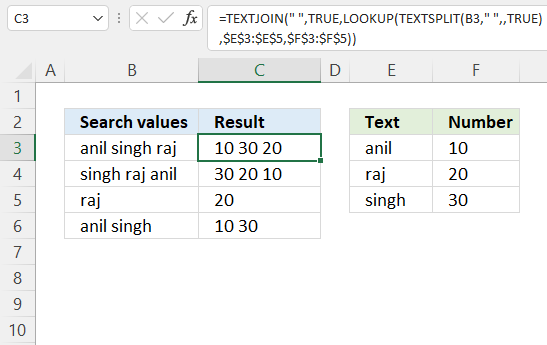Excel 365 formula in cell C3:

=TEXTJOIN(" ", TRUE, LOOKUP(TEXTSPLIT(B3, " ", , TRUE), \$E\$3:\$E\$5, \$F\$3:\$F\$5))

### Explaining formula

#### Step 1 - Split value into substrings

The TEXTSPLIT function splits a string into an array based on delimiting values.

Function syntax: TEXTSPLIT(Input_Text, col_delimiter, [row_delimiter], [Ignore_Empty])

TEXTSPLIT(B3, " ", , TRUE)

becomes

TEXTSPLIT("anil singh raj", " ", , TRUE)

and returns {"anil","singh","raj"}.

#### Step 2 - Match values and return the corresponding values

The LOOKUP function find a value in a cell range and return a corresponding value on the same row.

Function syntax: LOOKUP(lookup_value, lookup_vector, [result_vector])

LOOKUP(TEXTSPLIT(B3, " ", , TRUE), \$E\$3:\$E\$5, \$F\$3:\$F\$5)

becomes

LOOKUP({"anil","singh","raj"},{"anil";"raj";"singh"},{10;20;30})

and returns {10,30,20}.

#### Step 3 - Join the corresponding values

The TEXTJOIN function combines text strings from multiple cell ranges.

Function syntax: TEXTJOIN(delimiter, ignore_empty, text1, [text2], ...)

TEXTJOIN(" ", TRUE, LOOKUP(TEXTSPLIT(B3, " ", , TRUE), \$E\$3:\$E\$5, \$F\$3:\$F\$5))

becomes

TEXTJOIN(" ", TRUE, {10,30,20})

and returns "10 30 20".